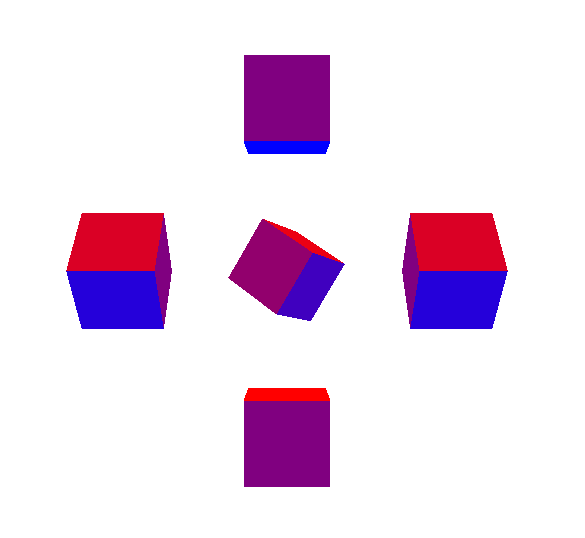three.js入门（3）—— 相机旋转功能、各种光源探究

//旋转相机以观察物体的不同角度
var cameraRound = 10;
$("body").bind("mousedown",cameraMove); var arf = 0; var oldArf = 0; function cameraMove(ev){ var mouseDownY = ev.pageY; var mouseDownX = ev.pageX; var zeroPoint = new THREE.Vector3(0, 0, 0);$("body").bind("mousemove",function(ev){
var mouseMoveY = ev.pageY;
var mouseMoveX = ev.pageX;
arf = (mouseMoveX - mouseDownX)/100+oldArf;
camera.position.z = cameraRound *(Math.cos(arf));
camera.position.x = cameraRound *(Math.sin(arf));
camera.lookAt(zeroPoint);
});
$("body").bind("mouseup",function(ev){$("body").unbind("mousemove");
oldArf = arf;
})
}

var material = new THREE.MeshLambertMaterial({color:0xffffff}); //使用Lambert材质

1.环境光（AmbientLight）

//环境光
var light = new THREE.AmbientLight(0xff00000);
scene.add(light);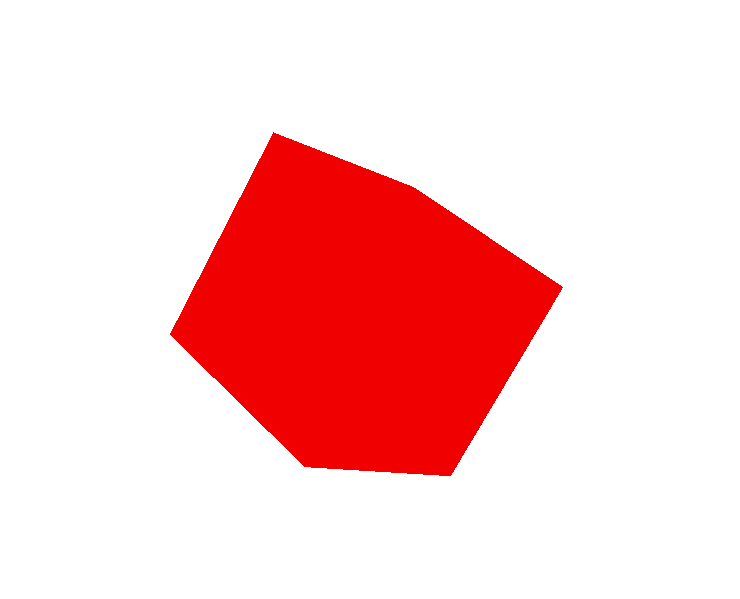var light = new THREE.AmbientLight(0xff00000);
var light2 = new THREE.AmbientLight(0x0000ff);
scene.add(light2);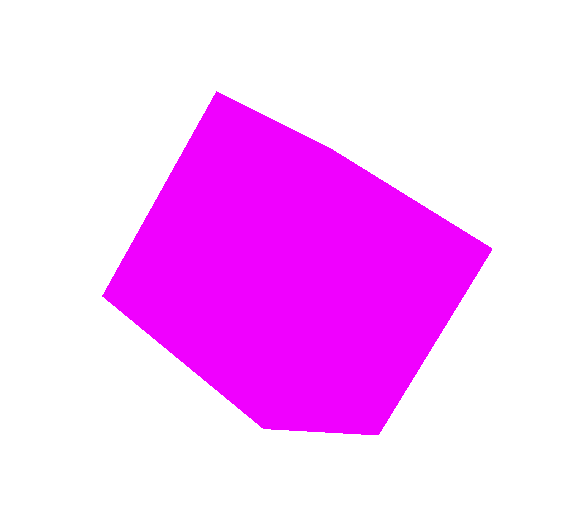//平行光
var light =  new THREE.DirectionalLight(0xff0000,1);
light.position.set(0,10,5);
//light.target=cube;
cube.position.set(-1,-1,2);//注意这里物体的位置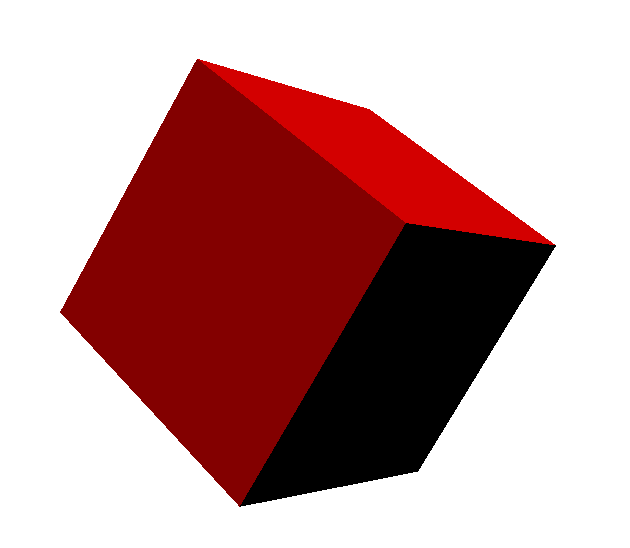//玩着玩着下班了，溜了溜了，下礼拜补。会去享受休息时光了。有什么问题可以一起交流的，打我电话，110.

hex -- 光源颜色的RGB数值。
intensity -- 光源强度的数值。(0-1)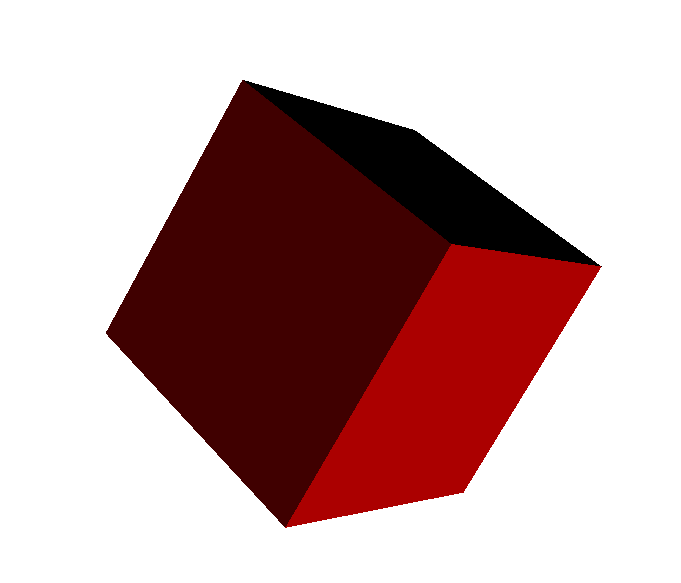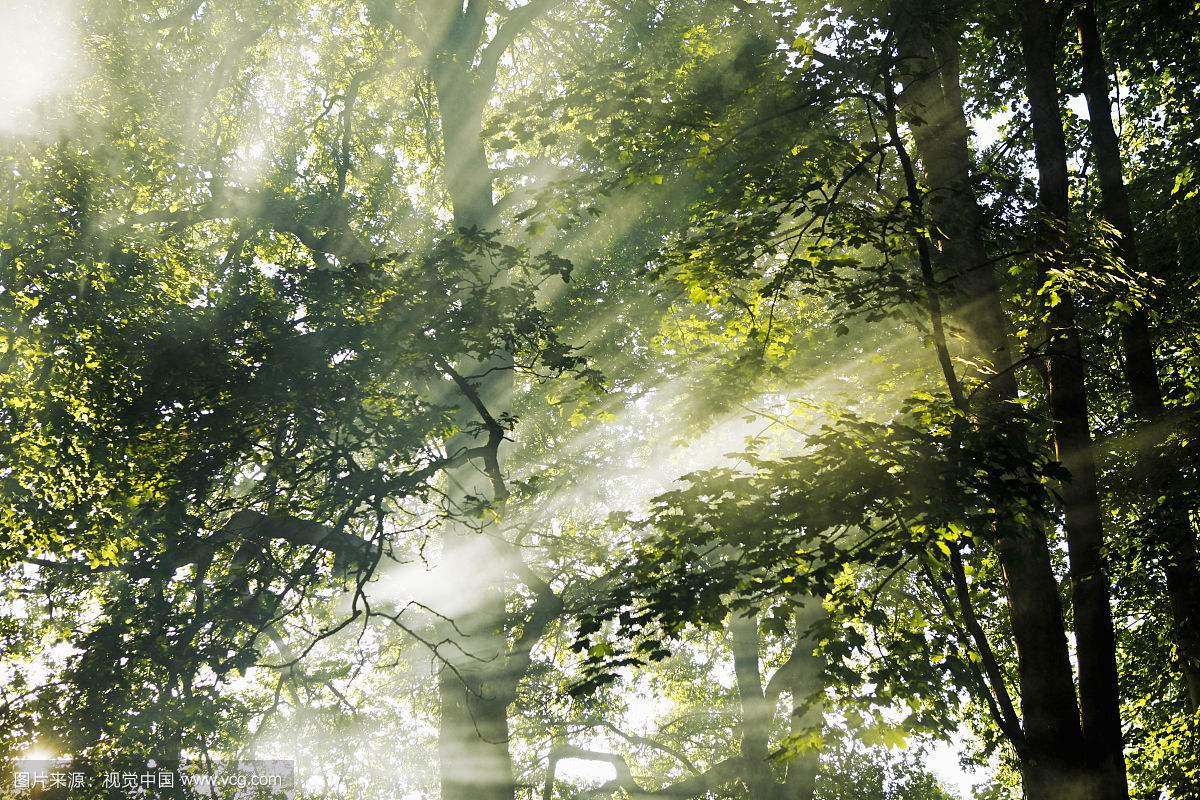//平行光
var light =  new THREE.DirectionalLight(0xff0000,1);
light.position.set(0,-10,5);
light.target=cube;
var light2 = new THREE.DirectionalLight(0x00ff00,1);
light2.target=cube;
light2.position.set(0,10,5);
var light3 = new THREE.DirectionalLight(0x0000ff,1);
light3.target=cube;
light3.position.set(-5,10,5);
scene.add(light3);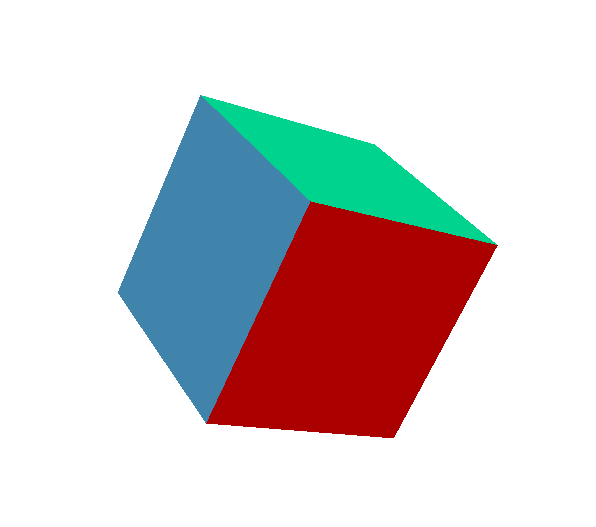var cube2 =  new THREE.Mesh(geometry,material);
var cube3 =  new THREE.Mesh(geometry,material);
var cube4 =  new THREE.Mesh(geometry,material);
var cube5 =  new THREE.Mesh(geometry,material);
cube.position.set(0,0,-4);
cube2.position.set(4,0,0);
cube3.position.set(0,4,0);
cube4.position.set(-4,0,0);
cube5.position.set(0,-4,0);
//点光源
var light = new THREE.PointLight( 0xff0000, 1, 100 );
light.position.set( 0, 0, 0 );
scene.add( light );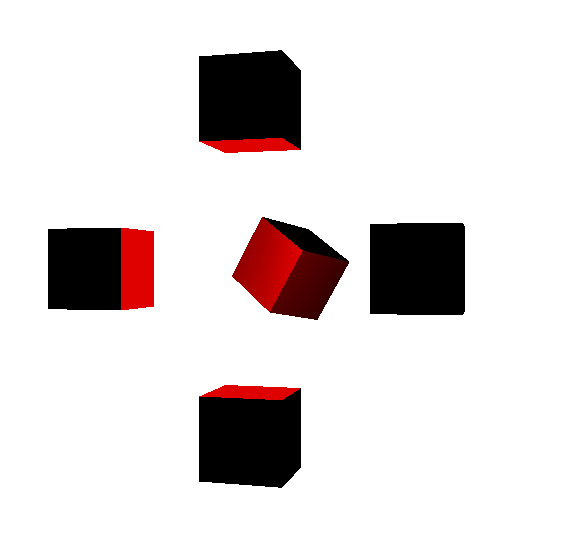PointLight( color, intensity, distance, decay )
color — 颜色的RBG数值。
intensity — 光强的数值。
distance -- 光强为0处到光源的距离，0表示无穷大。
decay -- 沿着光照距离的衰退量。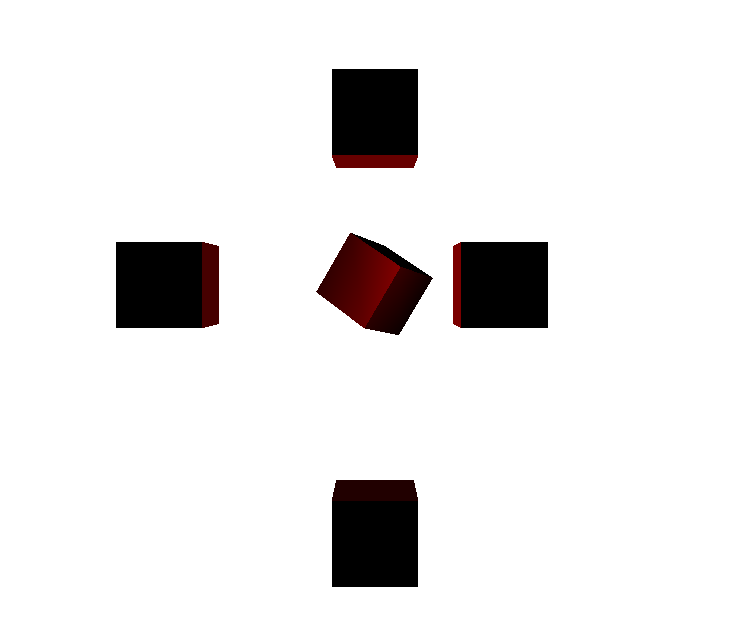.decay 沿着光照距离的衰退量。

//半球光源
var light = new THREE.HemisphereLight( 0xff0000, 0x0000ff, 1 );
scene.add( light );